# Capacitor & Capacitance Notes | Study Physics For JEE - JEE

## JEE: Capacitor & Capacitance Notes | Study Physics For JEE - JEE

The document Capacitor & Capacitance Notes | Study Physics For JEE - JEE is a part of the JEE Course Physics For JEE.
All you need of JEE at this link: JEE

What is a Capacitor?

• A capacitor is a two-terminal electrical device that possesses the ability to store energy in the form of an electric charge. It consists of two electrical conductors that are separated by a distance.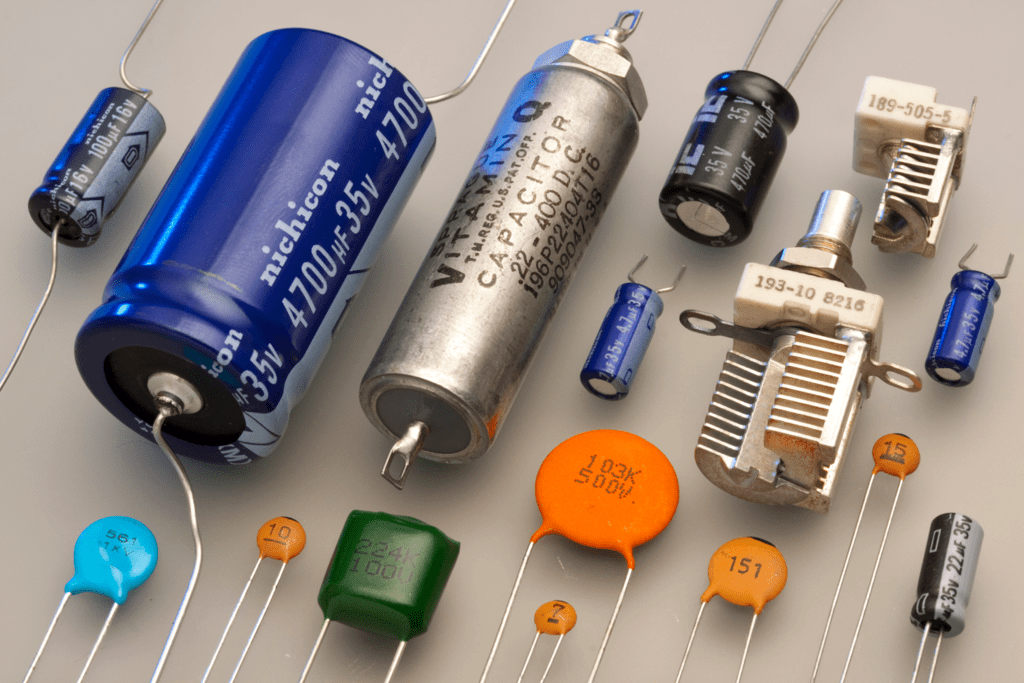Different Types of Capacitor
• The space between the conductors may be filled by vacuum or with an insulating material known as a dielectric. The ability of the capacitor to store charges is known as capacitance.
• Capacitors store energy by holding apart pairs of opposite charges. The simplest design for a capacitor is a parallel plate, which consists of two metal plates with a gap between them. But, there are different types of capacitors manufactured in many forms, styles, lengths, girths, and many materials.

How Does a Capacitor Work?

• For demonstration, let us consider the most basic structure of a capacitor – the parallel plate capacitor. It consists of two parallel plates separated by a dielectric. When we connect a DC voltage source across the capacitor, one plate is connected to the positive end (plate I) and the other plate to the negative end (plate II).
• When the potential of the battery is applied across the capacitor, plate I become positive with respect to plate II. At the steady-state condition, the current tries to flow through the capacitor from its positive plate to its negative plate. But it is unable to flow due to the separation of these with an insulating material.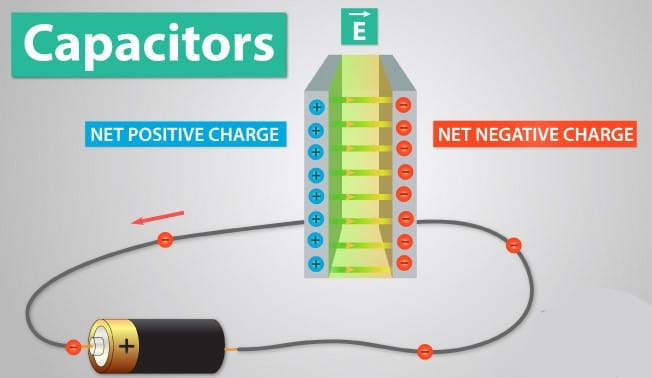How Does a Capacitor Work?
• An electric field appears across the capacitor. The positive plate (plate I) accumulates positive charges from the battery, and the negative plate (plate II) will accumulate negative charges from the battery. After a point, the capacitor holds the maximum amount of charge as per its capacitance with respect to this voltage. This time span is called the charging time of the capacitor.
• When the battery is removed from the capacitor, the two plates hold a negative and positive charge for a certain time. Thus, the capacitor acts as a source of electrical energy.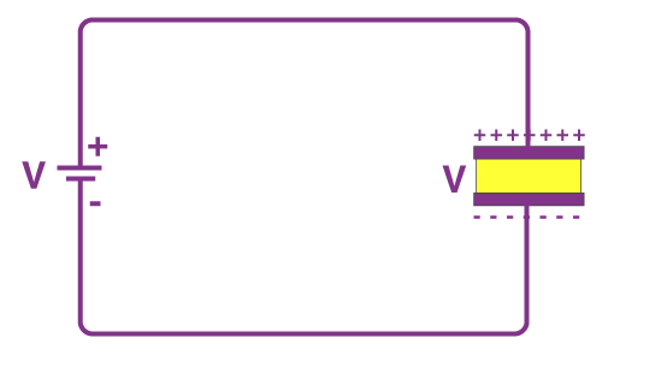• If these plates are connected to a load, the current flows to the load from Plate I to Plate II until all the charges are dissipated from both plates. This time span is known as the discharging time of the capacitor.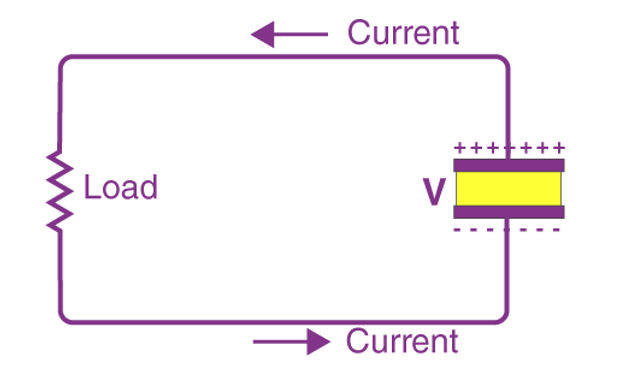How do you Determine the Value of Capacitance?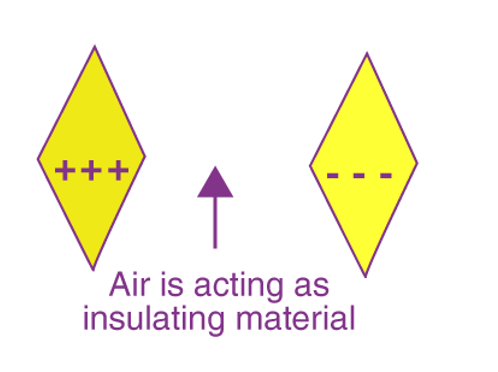• The conducting plates have some charges q1 and q2 (Usually if one plate has +q the other has –q charge). The electric field in the region between the plates depends on the charge given to the conducting plates. We also know that potential difference (V) is directly proportional to the electric field hence we can say,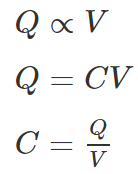• This constant of proportionality is known as the capacitance of the capacitor.
• Capacitance is the ratio of the change in the electric charge of a system, to the corresponding change in its electric potential.
• The capacitance of any capacitor can be either fixed or variable depending on their usage. From the equation, it may seem that ‘C’ depends on charge and voltage. Actually, it depends on the shape and size of the capacitor and also on the insulator used between the conducting plates.

Energy Stored in a Capacitor

• Once the opposite charges have been placed on either side of a parallel-plate capacitor, the charges can be used to do work by allowing them to move towards each other through a circuit. The total energy that can be extracted from a fully charged capacitor is given by the equation: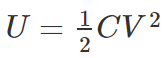• Capacitors function a lot of like rechargeable batteries. The main difference between a capacitor and a battery lies in the technique they employ to store energy. Unlike batteries, the capacitor’s ability to store energy doesn’t come from chemical reactions, rather from the physical design that allows it to hold negative and positive charges apart.

Standard Units of Capacitance

Most of the electrical and electronic applications are covered by the following standard unit (SI) prefixes for easy calculations:

• 1 mF (millifarad) = 10−3 F
• 1 μF (microfarad) =10−6 F
• 1 nF (nanofarad) = 10−9 F
• 1 pF (picofarad) = 10−12 F

Different Types of Capacitor

1. Capacitance of a Parallel Plate Capacitor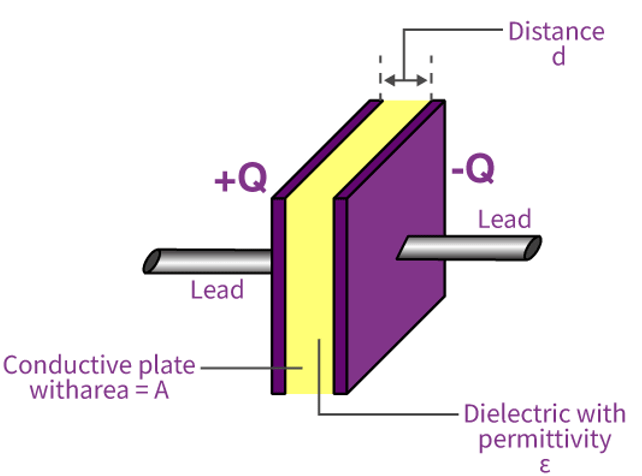• The parallel plate capacitor as shown in the figure has two identical conducting plates, each having a surface area A and separated by a distance d. When voltage V is applied to the plates, it stores charge Q.
• The force between charges increases with charge values and decreases with the distance between them. The bigger the area of the plates, the more charge they can store.  Hence, the value of C is greater for a large value of A. Similarly, the closer the plates are, the greater the attraction of the opposite charges on them. Therefore C is greater for a smaller d.
• The charge density on the plates is given by the formula: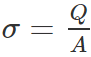• When the distance of separation (d) is small, the electric field between the plates is fairly uniform and its magnitude is given by: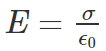• As the electric field between the plates is uniform, the potential difference between the plates is given by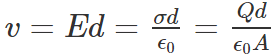• Substituting the above value of V in the capacitance formula, we get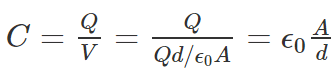• The capacitance of a parallel plate capacitor is given by the formula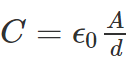Example. Calculate the capacitance of an empty parallel-plate capacitor that has metal plates with an area of 1.00 m2, separated by 1.00 mm?
Solution: Using the formula, we can calculate the capacitance as follows: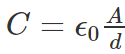Substituting the values, we get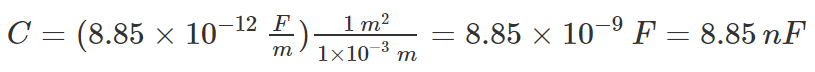2. Capacitance of a Spherical Capacitor

• Spherical capacitors consist of two concentric conducting spherical shells of radii R1 and R2. The shells are given equal and opposite charges +Q and –Q respectively. The electric field between shells is directed radially outward.
• The magnitude of the field can be obtained by applying Gauss law over a spherical Gaussian surface of radius r concentric with the shells.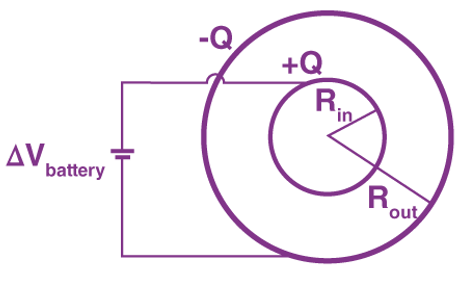• The enclosed charge is +Q, therefore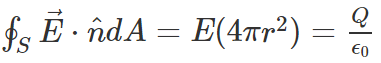• The electric field between the conductor is given as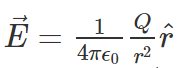• Integrating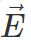along the radial path between the shells, we get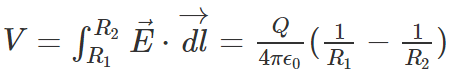• The potential difference between two conductors can be calculated using the formula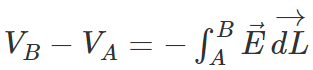• The potential difference between the plates is
V = - (V2 - V1) = V1 - V2
• Substituting the value of V in the capacitance formula, we get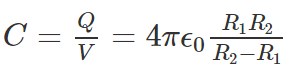• The capacitance of a spherical capacitor is given by the equation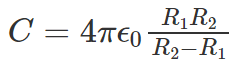Example. A spherical capacitor has an inner sphere of radius 12 cm and an outer sphere of radius 13 cm. The outer sphere is earthed and the inner sphere is given a charge of 2.5 µC. The space between the concentric spheres is filled with a liquid of dielectric constant 32. Determine the capacitance of the capacitor.
Solution:

Given:
The radius of the inner sphere,  r2 = 12 cm = 0.12 m
The radius of the outer sphere, r1= 13 cm = 0.13 m
Charge on the inner sphere, q = 2.5 μC = 2.5 x 10-6 C
Dielectric constant of a liquid, ∈r = 32
The capacitance of a spherical capacitor is given by the relation: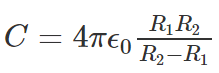0 = Permittivity of free space = 8.85 x 10-12 C2 N-1 m-2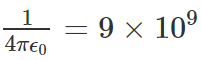Substituting the values in the equation, we get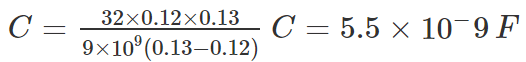3. Cylindrical Capacitor

• Cylindrical capacitor consists of two co-axial cylinders of radii a and b and length l. If a charge q is given to the inner cylinder, induced change -q will reach the inner surface of the outer cylinder. By symmetry, the electric field in region between the cylinders is radially outwards.
• By Gauss's theorem, the electric field at a distance r from the axis of the cylinder is given by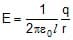• The potential difference between the cylinders is given by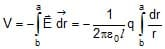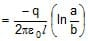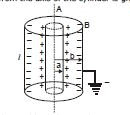or,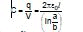Factors Affecting Capacitance

Dielectric

• The effect of dielectric on capacitance is that the greater the permittivity of the dielectric the greater the capacitance, likewise lesser the permittivity of the dielectric the lesser is the capacitance. Some materials offer less opposition to the field flux for a given amount of field force. Materials with greater permittivity allow more field flux, hence greater charge is collected.

Plate Spacing

• The effect of spacing on the capacitance is that it is inversely proportional to the distance between the plates. Mathematically it is given as: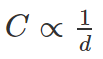Area of the Plates

• The effect of the area of the plate is that the capacitance is directly proportional to the area. Larger the plate area more is the capacitance value. Mathematically it is given as:
C ∝ A

What are the Applications of Capacitors?

➢ Capacitors for Energy Storage

• Since the late 18th century, capacitors are used to store electrical energy. Individual capacitors do not hold a great deal of energy, providing only enough power for electronic devices to use during temporary power outages or when they need additional power.
• There are many applications that use capacitors as energy sources and a few of them are as follows:
(i) Audio equipment
(ii) Camera Flashes
(iii) Power supplies
(iv) Magnetic coils
(v) Lasers
• Supercapacitors are capacitors that have high capacitances up to 2 kF. These capacitors store large amounts of energy and offer new technological possibilities in areas such as electric cars, regenerative braking in the automotive industry and industrial electrical motors, computer memory backup during power loss, and many others.

➢ Capacitors for Power Conditioning

• One of the important applications of capacitors is the conditioning of power supplies. Capacitors allow only AC signals to pass when they are charged blocking DC signals.
• This effect of a capacitor is majorly used in separating or decoupling different parts of electrical circuits to reduce noise, as a result of improving efficiency. Capacitors are also used in utility substations to counteract inductive loading introduced by transmission lines.

➢ Capacitors as Sensors

• Capacitors are used as sensors to measure a variety of things including humidity, mechanical strain, and fuel levels. Two aspects of capacitor construction are used in the sensing application – the distance between the parallel plates and the material between them.
• The former is used to detect mechanical changes such as acceleration and pressure and the latter is used in sensing air humidity.

➢ Capacitors for Signal Processing

• There are advanced applications of capacitors in information technology. Capacitors are used by Dynamic Random Access Memory (DRAM) devices to represent binary information as bits.
• Capacitors are also used in conjunction with inductors to tune circuits to particular frequencies, an effect exploited by radio receivers, speakers, and analog equalizers.

Frequently Asked Questions on Capacitors and Capacitance

Q.1. What is a variable capacitor?
Ans. A variable capacitor is a capacitor whose capacitance can be varied to a certain range of values based on the necessity. The two plates of the variable capacitor are made of metals where one of the plates is fixed and the other plate is movable. Their main function is to fix the resonant frequency in the LC circuit. There are two types of variable frequency and they are, tunning capacitors and trimming capacitors.

Q.2. How does the shape of the capacitor affect its capacitance?
Ans. The distance between the plates:

• The more distant the plates are, the less the free electrons on the far plate feel the push of the electrons that are being added to the negative plate. This makes it harder to add more negative charges to the negative plate. If the plates are closer to each other, the current would flow through a short circuit. This implies that the capacitance of a parallel plate is inversely related to the plate separation.

Area of the plates:

• It’s a lot easier to add charges to a capacitor if the parallel plates have a huge area. Two wide metal plates would give two repelling like charges a greater range to spread out across the plate, making it easier to add a lot more negative charge to one plate. Likewise, a very small plate area would cause the electrons to get cramped together earlier, making it harder to get a large difference in charge for a given voltage.

Q.3. What are Ultracapacitors?
Ans. An ultracapacitor, also known as the supercapacitor, is a high-capacity capacitor with a capacitance value much higher than other capacitors, but with lower voltage limits.

Q.4. How long does a capacitor last?
Ans. Capacitors have a limited life span. Most capacitors are designed to last approximately 20 years.

Q.5. What kind of energy is stored in a capacitor?
Ans. Energy stored in a capacitor is electrical potential energy, and it is thus related to the charge Q and voltage V on the capacitor.

Q.6. Why isn’t water used as a dielectric in a capacitor?
Ans. Water has a high dielectric constant but a very low dielectric strength, hence it would act as a conductor and would leak charges through it.

The document Capacitor & Capacitance Notes | Study Physics For JEE - JEE is a part of the JEE Course Physics For JEE.
All you need of JEE at this link: JEEUse Code STAYHOME200 and get INR 200 additional OFF

## Physics For JEE

196 videos|531 docs|256 tests

Track your progress, build streaks, highlight & save important lessons and more!

,

,

,

,

,

,

,

,

,

,

,

,

,

,

,

,

,

,

,

,

,

;